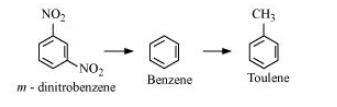# Out of benzene, m–dinitrobenzene and toluene

Question:

Out of benzene, m–dinitrobenzene and toluene which will undergo nitration most easily and why?

Solution:

The ease of nitration depends on the presence of electron density on the compound to form nitrates. Nitration reactions are examples of electrophilic substitution reactions where an electron-ich species is attacked by a nitronium ion $\left(\mathrm{NO}_{2}-\right)$.

Now, $\mathrm{CH}_{3}-$ group is electron donating and $\mathrm{NO}_{2}-$ is electron withdrawing. Therefore, toluene will have the maximum electron density among the three compounds followed by benzene. On the other hand, m- Dinitrobenzene will have the least electron density. Hence, it will undergo nitration with difficulty. Hence, the increasing order of nitration is as follows: